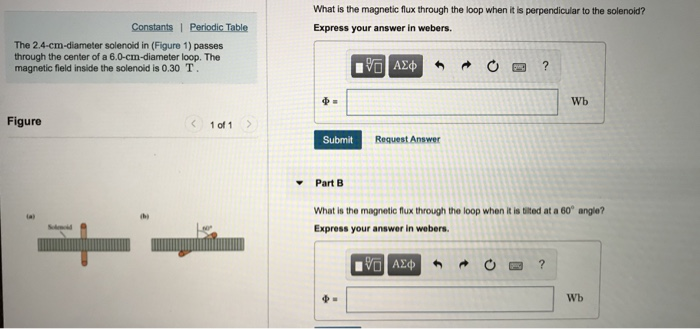# Problem: The 2.4-cm-diameter solenoid in (Figure 1) passes through the center of a 6.0-cm-diameter loop.  The magnetic field inside the solenoid is 0.30 T.Part A What is the magnetic flux through the loop when it is perpendicular to the solenoid? Express your answer in webers. Part B What is the magnetic flux through the loop when it is tilted at a 60 angle? Express your answer in webers.

###### FREE Expert Solution

Magnetic flux:

$\overline{){{\mathbf{\varphi }}}_{{\mathbf{B}}}{\mathbf{=}}{\mathbf{B}}{\mathbf{A}}{\mathbf{c}}{\mathbf{o}}{\mathbf{s}}{\mathbf{\theta }}}$

The cross-sectional area of the solenoid:

A = πr2

r = 2.4/2 = 1.2 cm

A = (π)(1.2 × 10-2)2 = 4.524 × 10-4  m2

90% (238 ratings)###### Problem Details

The 2.4-cm-diameter solenoid in (Figure 1) passes through the center of a 6.0-cm-diameter loop.  The magnetic field inside the solenoid is 0.30 T.

Part A What is the magnetic flux through the loop when it is perpendicular to the solenoid? Express your answer in webers.Part B What is the magnetic flux through the loop when it is tilted at a 60 angle? Express your answer in webers.